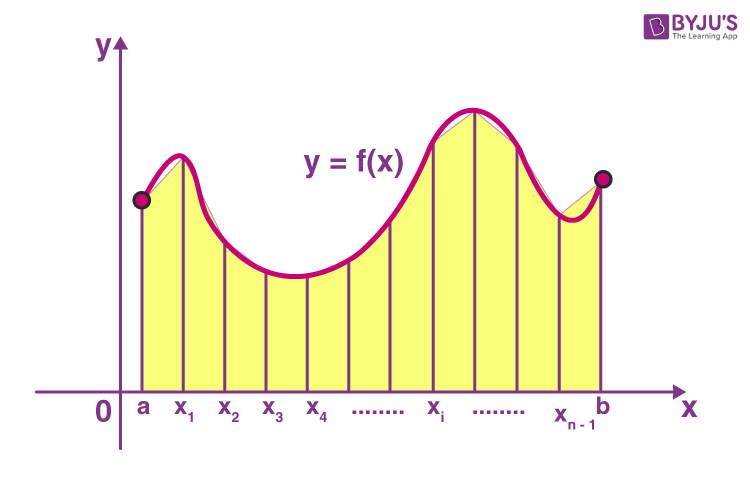# Trapezoidal Rule

In Calculus, “Trapezoidal Rule” is one of the important integration rules. The name trapezoidal is because when the area under the curve is evaluated, then the total area is divided into small trapezoids instead of rectangles. This rule is used for approximating the definite integrals where it uses the linear approximations of the functions.

The trapezoidal rule is mostly used in the numerical analysis process. To evaluate the definite integrals, we can also use Riemann Sums, where we use small rectangles to evaluate the area under the curve.

## Trapezoidal Rule DefinitionTrapezoidal Rule is a rule that evaluates the area under the curves by dividing the total area into smaller trapezoids rather than using rectangles. This integration works by approximating the region under the graph of a function as a trapezoid, and it calculates the area. This rule takes the average of the left and the right sum.

The Trapezoidal Rule does not give accurate value as Simpson’s Rule when the underlying function is smooth. It is because Simpson’s Rule uses the quadratic approximation instead of linear approximation. Both Simpson’s Rule and Trapezoidal Rule give the approximation value, but Simpson’s Rule results in even more accurate approximation value of the integrals.

## Trapezoidal Rule Formula

Let f(x) be a continuous function on the interval [a, b]. Now divide the intervals [a, b] into n equal subintervals with each of width,

Δx = (b-a)/n, Such that a = x0 < x1< x2< x3<…..<xn = b

Then the Trapezoidal Rule formula for area approximating the definite integral ∫ab f(x)dx is given by:

$$\begin{array}{l}\int_{a}^{b}f(x)dx\approx T_{n}=\frac{\bigtriangleup x}{2}[f(x_{0})+ 2f(x_{1})+2f(x_{2})+….2f(x_{n-1})+f(x_{n})]\end{array}$$

Where, xi = a+iΔx

If n →∞, R.H.S of the expression approaches the definite integral ∫ab f(x)dx.

## Solved Examples

Go through the below given Trapezoidal Rule example.

Example 1:

Approximate the area under the curve y = f(x) between x =0 and x=8 using Trapezoidal Rule with n = 4 subintervals. A function f(x) is given in the table of values.

 x 0 2 4 6 8 f(x) 3 7 11 9 3

Solution:

The Trapezoidal Rule formula for n= 4 subintervals is given as:

T4 =(Δx/2)[f(x0)+ 2f(x1)+ 2f(x2)+2f(x3) + f(x4)]

Here the subinterval width Δx = 2.

Now, substitute the values from the table, to find the approximate value of the area under the curve.

A≈ T4 =(2/2)[3+ 2(7)+ 2(11)+2(9) + 3]

A≈ T4 = 3 + 14 + 22+ 18+3 = 60

Therefore, the approximate value of area under the curve using Trapezoidal Rule is 60.

Example 2:

Approximate the area under the curve y = f(x) between x =-4 and x= 2 using Trapezoidal Rule with n = 6 subintervals. A function f(x) is given in the table of values.

 x -4 -3 -2 -1 0 1 2 f(x) 0 4 5 3 10 11 2

Solution:

The Trapezoidal Rule formula for n= 6 subintervals is given as:

T6 =(Δx/2)[f(x0)+ 2f(x1)+ 2f(x2)+2f(x3) + 2f(x4)+2f(x5)+ f(x6)]

Here the subinterval width Δx = 1.

Now, substitute the values from the table, to find the approximate value of the area under the curve.

A≈ T6 =(1/2)[0+ 2(4)+ 2(5)+2(3) + 2(10)+2(11) +2]

A≈ T6 =(½) [ 8 + 10 + 6+ 20 +22 +2 ] = 68/2 = 34

Therefore, the approximate value of area under the curve using Trapezoidal Rule is 34.

Register with BYJU’S – The Learning App to read all Calculus related topics and download the App to watch interactive videos.

## Frequently Asked Questions – FAQs

Q1

### What is Trapezoidal Rule?

Trapezoidal Rule is an integration rule, in Calculus, that evaluates the area under the curves by dividing the total area into smaller trapezoids rather than using rectangles.
Q2

### Why the rule is named after trapezoid?

The name trapezoidal is because when the area under the curve is evaluated, then the total area is divided into small trapezoids instead of rectangles. Then we find the area of these small trapezoids in a definite interval.
Q3

### What is the difference between Trapezoidal rule and Riemann Sums rule?

In trapezoidal rule, we use trapezoids to approximate the area under the curve whereas in Riemann sums we use rectangles to find area under the curve, in case of integration.